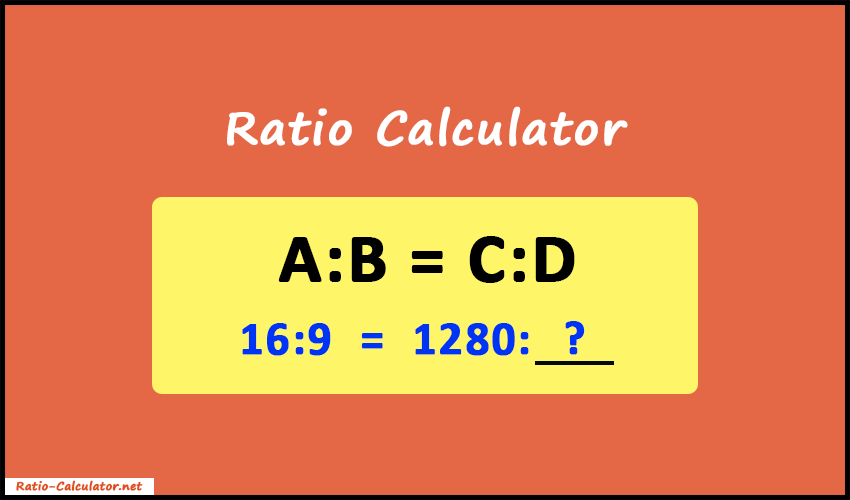# Ratio Calculator

The ratio calculator allows you to compare the ratios and find one missing value from three known numbers.

##### More Useful Tools
Fraction Calculator GCF Calculator LCM Calculator

Ratio Calculator is a free online tool where you can compare two ratios and find one missing value. The main operation of the calculator is to solve the ratios using the three values provided by the user and find one missing value. Also, it helps you to find the aspect ratio of two sizes or objects.## How to use the Ratio Calculator?

Our calculator is very simple to use. But there are some important rules you need to keep in mind.

Necessary Rules:

• Firstly, you have to enter any of the three input boxes and leave one empty that you want to find.
• You can only input integer and decimal numbers. No alphabets are allowed. Even negative and fraction values are not supported.
• No special characters are allowed except the dot(.) symbol for decimal value.

Now follow the steps below to use the ratio calculator.

1. Start entering the values inside the input boxes arranged in the form of an equation. Make sure, your value resides in the correct position. Leave the box vacant whose value is to be calculated.
2. After entering the ratios, tap the Calculate button.
3. As a result, you will get the missing value along with calculation steps and formula.
4. For a new calculation just press the Reset button.

### What is Ratio?

The ratio can be simply defined as the mathematical relationship between two numbers that are used to express the proportion of the first number containing the second number.

We can write the ratios in following terms:

15:7 or 15 to 7 or 15/7

Let's understand it using an example. In a school, it is said that for every 50 students there is 1 teacher. Therefore, the ratio will be 50:1 between students and teachers. So, if the number of students is 150 so there would be 3 teachers.

Ratios are also used to show the interrelation between two sizes. There can be more complex ratios depending upon the use cases. For example, for every 50 students, there is 1 teacher and 2 staff members, so the ratio will come out as 50:1:2.

The ratio is dimensionless. So, it doesn't have any units. Also, it is widely used to compare the width and height of any object. Like screen sizes and video resolutions. In addition, it is used in business metrics, science, decision-making strategies, accountancy, statistics, etc.

### How to Calculate the Ratio?

It's obvious that our Ratio Calculator is the best tool that will help you to calculate the missing part to make both the ratios equivalent. Now let's understand the mathematics used behind the calculator.

The proportion equation we have:

A : B = C : D

or, A / B = C / D (in fraction)

or, A × D = C × B (by cross multiplication)

Now, we can easily manipulate the above equation to solve for the one value we need.

If we need to find the value of D and we have A, B, and C, then the equation will be:

D =
 C × B A

Similarly, the equations for the other values are:

A =
 C × B D
B =
 A × D C
C =
 A × D B

### Ratio Calculation Process

The step-by-step process to solve for an unknown value is as follows:

1. Firstly, write the ratio values into fractions.
2. Do a simple cross multiplication. Multiply the numerator of the left-hand side fraction with the denominator of the right-hand side fraction. Similarly, multiply the denominator of the left-hand side fraction with the numerator of the right-hand side fraction.
3. Now, keep the unknown variable on one side and move the single known variable to the denominator of the other side.

Let's understand the above process with an example.

##### Example:

12 : 18 = C : 5
Find the missing value C.

##### Solution:

12 : 18 = C : 5

Firstly, let's convert the ratios into fractions.

 12 18
=
 C 5
C =
 A × D B
=
 12 × 5 18
=
 60 18

So, C = 3.333

Hence, the missing value in the equation is 3.333 and it makes both ratios equivalent.